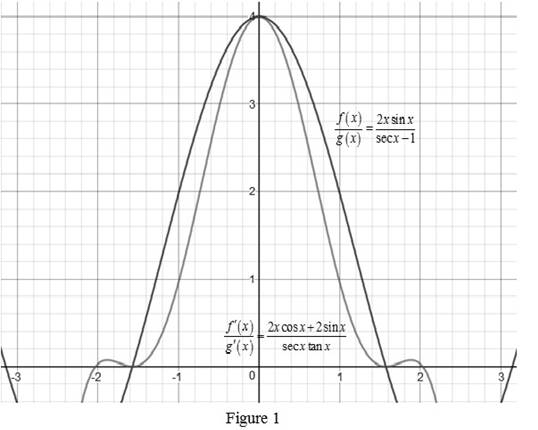# To illustrate: L’Hospitals rule by ploting f ( x ) g ( x ) and f ′ ( x ) g ′ ( x ) near x = 0 to check whether the limits are the same and calculate the exact value of the limit.### Single Variable Calculus: Concepts...

4th Edition
James Stewart
Publisher: Cengage Learning
ISBN: 9781337687805### Single Variable Calculus: Concepts...

4th Edition
James Stewart
Publisher: Cengage Learning
ISBN: 9781337687805

#### Solutions

Chapter 4.5, Problem 50E
To determine

## To illustrate: L’Hospitals rule by ploting f(x)g(x) and f′(x)g′(x) near x=0 to check whether the limits are the same and calculate the exact value of the limit.

Expert Solution

The exact value of limx02xsinxsecx1 is 4.

### Explanation of Solution

Given:

The functions are f(x)=2xsinx and g(x)=secx1 .

Calculation:

The value of f(x)g(x)=2xsinxsecx1 .

The value of, f(x)g(x)=2xcosx+2sinxsecxtanx .

Use the online graphing calculator and draw the graphs of f(x)g(x)=2xsinxsecx1 and f(x)g(x)=2xcosx+2sinxsecxtanx on the same plane as shown below in Figure 1.From Figure 1, it is identified that both curves approach to 4 as x approaches 0. Thus, f(x)g(x) and f(x)g(x) approaches the same limit.

Find the exact limit with the help of L’Hospital’s rule as follows.

Obtain the value of the function as x approaches 0 .

As x approaches 0, the numerator is,

2xsinx=20sin0=0

As x approaches 0, the denominator is,

secx1=sec01=11=0 .

Thus, limx02xsinxsecx1=00 is in an indeterminate form.

Therefore, apply L’Hospital’s Rule and obtain the limit.

limx02xsinxsecx1=limx02sinx+2xcosxsecxtanx

As x approaches 0, the numerator is,

2sinx+2xcosx=2sin0+20cos0=20+201=0+0=0

As x approaches 0, the denominator is,

secxtanx=sec0tan0=10=0

Thus, limx02sinx+2xcosxsecxtanx=00 is again in an indeterminate form.

Therefore, apply L’Hospital’s Rule again and obtain the limit.

limx02sinx+2xcosxsecxtanx=limx02cosx+2cosx2xsinxsec3x+secxtan2x=2cos0+2cos020sin0sec30+sec0tan20=2+201+10=4

Thus, limx02xsinxsecx1=4 .

Therefore, the exact value of limx02xsinxsecx1=4 .

### Have a homework question?

Subscribe to bartleby learn! Ask subject matter experts 30 homework questions each month. Plus, you’ll have access to millions of step-by-step textbook answers!